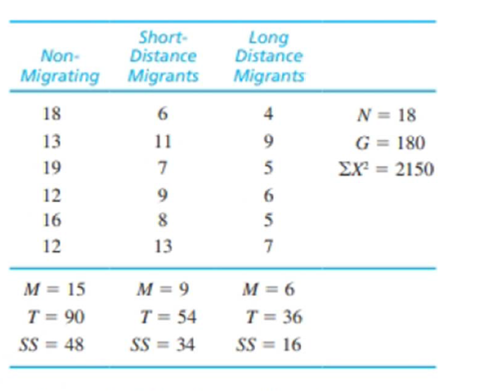Chapter 12, Problem 22PEssentials of Statistics for the B...

8th Edition
Frederick J Gravetter + 1 other
ISBN: 9781133956570

Solutions

Chapter
SectionEssentials of Statistics for the B...

8th Edition
Frederick J Gravetter + 1 other
ISBN: 9781133956570
Textbook Problem

One possible explanation for why some birds migrate and others maintain year round residency in a single location is intelligence. Specifically, birds with small brains, relative to their body size, are simply not smart enough to find food during the winter and must migrate to warmer climates where food is easily available (Sol. Lefebvre, & Rodriguez-Teijeiro, 2005). Birds with bigger brains, on the other hand, are more creative and can find food even when the weather turns harsh. Following are hypothetical data similar to the actual research results. The numbers represent relative brain size for the individual birds in each sample.a. Use an ANOVA with α = .05 to determine whether there are any significant mean differences among the three groups of birds.b. Compute η 2, the percentage of variance explained by the group differences, for these data.c. Write a sentence demonstrating how a research report would present the results of the hypothesis test and the measure of effect size.d. Use the Tukey HSD posttest to determine which groups are significantly different.

a.

To determine

To test: Whether there is any significant difference among the three groups.

Explanation

Given info:

One survey was done on the brain size of different types of bird.

 Non-migrating Short-Distance Migrants Long-Distance Migrants N=18G=180∑X2=2150 M=15 M=9 M=6 T=90 T=54 T=36 SS=48 SS=34 SS=16 n=6 n=6 n=6

Calculation:

The null and alternative hypotheses:

Null hypothesis:

H0:μ1=μ2=μ3.

That is, there is no significant difference between the means.

Alternate hypothesis:

Ha:atleast two means are different

Degrees of freedom:

It is known that for k number of treatments the degrees of freedom is,

df=k1.

Thus,

dfbetween=k1=31=2

Similarly,

dfwithin=Nk=183=15

Critical value:

For α=0.05 and df=10, the critical value is 3.68.

The sum of square due to total is SStotal=X2G2N, where G is total number of observations.

Thus,

SStotal=X2G2N=2150180218=350

And

SSwithin=SSinsidethetreatment&#

b.

To determine

To find: The value of η2

c.

To determine

To write: A sentence that demonstrate how a research report would present the results of the hypothesis test and the measure of effect size.

d.

To determine

To find: Which groups are significantly different using Tukey HSD posttest.

Still sussing out bartleby?

Check out a sample textbook solution.

See a sample solution

The Solution to Your Study Problems

Bartleby provides explanations to thousands of textbook problems written by our experts, many with advanced degrees!

Get Started